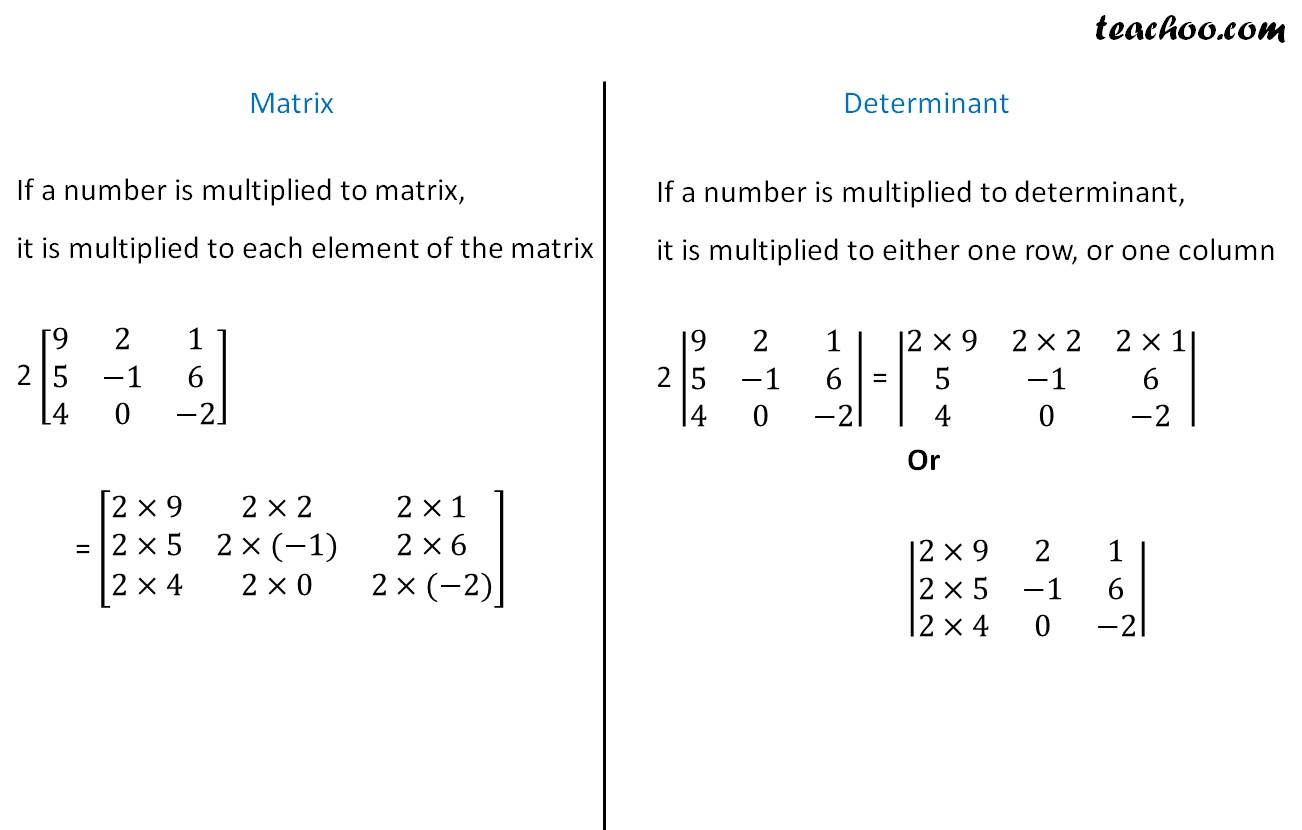Miscellaneous

Chapter 4 Class 12 Determinants
Serial order wise

Let’s look at various properties of Matrices and Determinants

## Addition and Subtraction of Matrices

• A + B = B + A
• (A + B) + C = A + (B + C)
• k (A + B) = kA + kB

## Multiplication of matrices

• AB ≠ BA
• (AB) C = A (BC)
• Distributive law
A (B + C) = AB + AC
(A + B) C = AC + BC
• Multiplicative identity
For a square matrix A
AI = IA = A

## Properties of transpose of matrix

• (A T ) T = A
• (kA) T = kA T
• (A + B) T = A T + B T
• (AB) T = B T A T

## Symmetric and Skew Symmetric matrices

• Symmetric Matrix - If A T = A

• Skew - symmetric Matrix - If A T = A
Note: In a skew matrix, diagonal elements are always 0 .

• For any square matrix A,
(A + A T ) is a symmetric matrix
(A − A T ) is a skew-symmetric matrix

## Inverse of a matrix

For a square matrix A, if

AB = BA = I

Then, B is the inverse of A

i.e. B = A −1

We will find inverse of a matrix by

## Properties of Inverse

1. For a matrix A,
A −1 is unique, i.e., there is only one inverse of a matrix

2. (A −1 ) −1 = A

3. (𝑘 𝐴) −1 = 1/𝑘 𝐴 −1
Note: This is different from
(kA) T = k A T
4. (A -1 ) T = (A T ) -1

5. (A + B) -1 = A -1 + B -1

6. (𝐴𝐵) −1 = 𝐵 −1 𝐴 −1

## Important things to note in Determinants

1. Determinant of Identity matrix = 1
det (I) = 1

2. |A T | = |A|

3. |AB| = |A| |B|

4. |A −1 | = 1/|𝐴|

5. |kA| = k n |A| where n is order of matrix

6. Similarly,
|−A| = |−1 × A|
= (−1) n × |A|

where n is the order of determinant

## Number multiplied to matrix and determinant## Other important points

Also, look at

Learn in your speed, with individual attention - Teachoo Maths 1-on-1 Class

### Transcript

Number multiplied to matrix and determinant Matrix If a number is multiplied to matrix, it is multiplied to each element of the matrix 2 [■8(9&2&1@5&−1&6@4&0&−2)] = [■8(2×9&2×2&2×1@2×5&2×(−1)&2×6@2×4&2×0&2×(−2))] Determinant If a number is multiplied to determinant, it is multiplied to either one row, or one column 2 |■8(9&2&1@5&−1&6@4&0&−2)| = |■8(2×9&2×2&2×1@5&−1&6@4&0&−2)| Or |■8(2×9&2&1@2×5&−1&6@2×4&0&−2)|